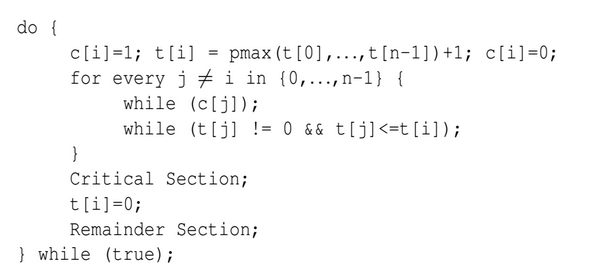Related Articles
GATE | GATE-CS-2016 (Set 1) | Question 60
• Difficulty Level : Expert
• Last Updated : 13 Feb, 2016

Consider the following proposed solution for the critical section problem. There are n processes: P0 …Pn−1. In the code, function pmax returns an integer not smaller than any of its arguments. For all i, t[i] is initialized to zero.Which one of the following is TRUE about the above solution?
(A) At most one process can be in the critical section at any time
(B) The bounded wait condition is satisfied
(C) The progress condition is satisfied
(D) It cannot cause a deadlock

Explanation:

```Mutual exclusion  is satisfied:
All other processes j started before i must have value (i.e. t[j])
less than the value of process i (i.e. t[i])  as function pMax()
return a integer not smaller  than any of its arguments. So if anyone
out of the processes j have positive value will be executing in its
critical section as long as the condition t[j] > 0 && t[j] <= t[i] within
while will persist. And when  this j process comes out of its critical
section, it sets t[j] = 0;  and next process will be selected in for loop.
So when i process reaches to its critical section none of the  processes j
which started earlier before process i  is in its critical section. This
ensure that only one process is executing its critical section at a time.
```
```Deadlock and progress are  not satisfied:
while (t[j] != 0 && t[j] <=t[i]); because of this condition deadlock is
possible when value of j process becomes equals to the value of process i
(i.e t[j] == t[i]).  because of the deadlock progess is also not possible
(i.e. Progess == no deadlock) as no one process is able to make progress
by stoping other process.
```
```Bounded waiting is also not satisfied:
In this case both deadlock and bounded waiting to be arising from the same
reason as if t[j] == t[i] is possible then starvation is possible means
infinite waiting.```

This explanation has been contributed by Dheerendra Singh.

Quiz of this Question

My Personal Notes arrow_drop_up